# The Bertrand Paradox: Understanding the logic, concept, and idea in a more approachable way(Edited)

If you thought the concept of Monty Hall paradox was complicated, permit me to inform you that, there is more in the bag. At first this concept was not looking too appetizing on first approach but when you analyze it deeply, you will be amazed how interesting it can. This is not rocket science, it is even something you can sit down and practicalize for yourself. The only that got me thinking was how Joseph Bertrand, a French Mathematician just woke up one faithful day to think about this. Either way, let's get to the point.

The Bertrand paradox is a paradox in theory of probability just like the Monty Hall we earlier discussed in our previous post. Just as how the Monty Hall was named after its founder, Bertrand paradox is no different. Here the whole concept is aimed at showing the dependence of the probability of an event on the the choice of a random process that is used to define the event. i.e. the chances of an event occurring, depends on the random experiment that characterized the event itself. You can as well put it this way, a problem that demonstrates the dependence of probability on the choice of a probability distribution (a function that describes the chances of an event to occur).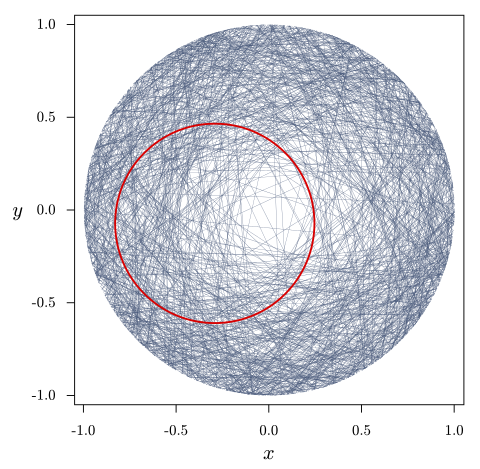So why is it considered a paradox?

One of the most interesting aspect of this concept is the fact that, it simply makes us understand that, those probability problems that are thought to be seemingly simple and well-defined, can at the long run have unexpected and counterintuitive solutions, and this happens when we do not clearly define the random experiment.

Now, because the random experiment is not well define, you end up having different results for a particular experiment. Bertrand using an equilateral triangle inscribed in a circle, was able to show that we could get different probability of outcomes for a chord drawn at any random point to be greater than the length of the inscribed triangle.

In essence, the paradox arises because the three different methods of selecting the chords randomly are never equivalent. When you apply each method, it turns that, it gives a different probability of outcome. This begs the question, what are these methods? We will address this questions shortly, but in the meantime, here is the actual question that led yielded the Bertrand paradox.

Consider an equilateral triangle that is inscribed in a circle. Suppose a chord of the circle is chosen at random. What is the probability that a random chord of circle is longer than a side of the triangle.

Personally when I saw the question, without thinking, I simply assumed that the problem should yield same result since it is an equilateral triangle inscribed in the circle. Secondly, I expected that the chords should be equal to the length of the triangle. Really, I was not just thinking straight at that point not until I understood better. At the onset, it was not just making any sense to me, but on the long run, they all made sense through personal studies and research.

Bertrand proposed three main ways that this probability can be solved and each method produced different results hence, the reason for the paradoxical nature of the question just like we earlier explained. To answer the question, three methods as proposed by Bertrand were the Random endpoint method, Random radial point and the Random midpoint method. Well, you will sure find some of the explanations of these concepts online, as they are not something new.

The only thing I cannot say for sure, is your understanding of the whole concept and various approaches used. The goal here is to make you understand everything that led to the results gotten from each methods. So let's get a deeper and better understanding, shall we? We will begin with the very first one.

The random endpoint method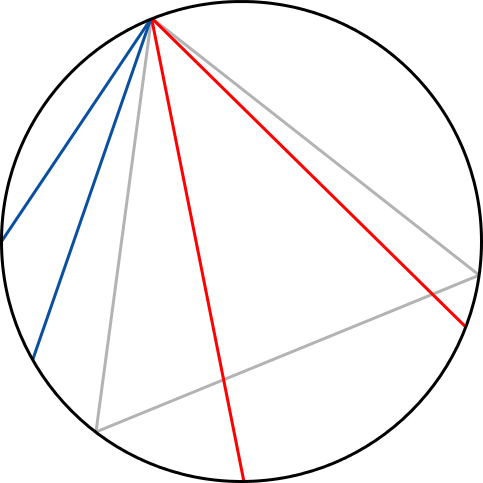Random chords, selection method 1; red = longer than triangle side, blue = shorter

At first, the concept may look difficult from afar, but when you approach it closely, you understand it better. I have made a better illustration to depict the whole concept for you to easily understand. Let us examine the image below.

When we consider that one end of the random chord drawn remains fixed at a point, say vertex A whiles its other ends - 1-6 is varying along the length of line BC. As long as the length of A1-6 is within or falls within that green shaded segment, then the length of the random chord is longer than the length of the sides of the inscribed circle.

You can as well practicalise this by using a ruler to measure the length of say line AP. You will sure notice that is the length of the chord AP is shorter than any of the chords A1-6 that are within the shaded region, hence, it is not longer than the length of the sides of the inscribed equilateral triangle. To find the probability that any of the length of the random chords A1-6 is longer than the length of the inscribed triangle, we could used different approach, but this method I will explain is much direct and easier.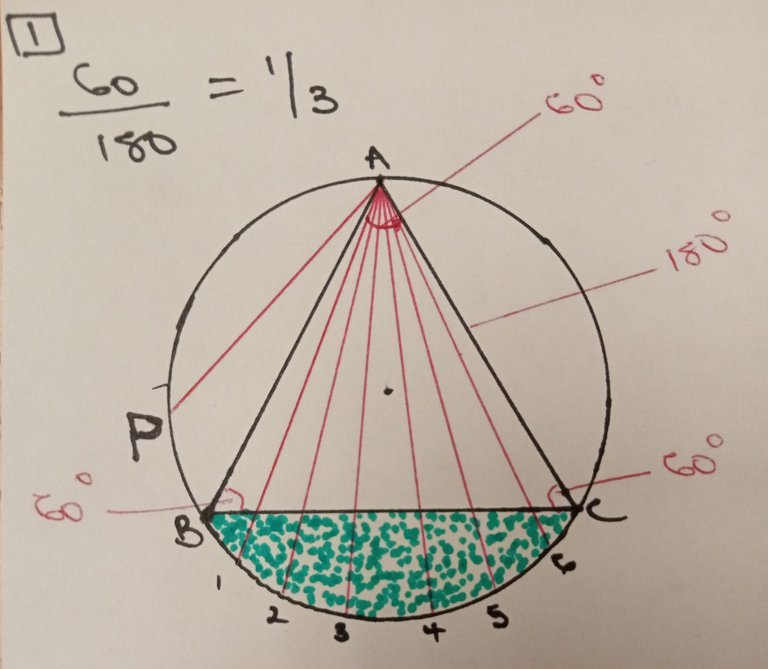Since we already know that the inscribed triangle is an equilateral triangle (has all its sides equal) and also we know that the total possible length of the sides of a triangle is 3, then the probability that the randomly drawn chord will longer than the length of the sides of the triangle is 1/3.

In a clearer terms, it is 1/3 because, the shaded segment only occupies one third of the whole circle as long as the vertex remains fixed. You can as well decide to change the vertex to be C, then it means that the new length of the chord would be AB. It does not matter the vertex you choose, there will always be only 1 segment occupied at once from a fixed vertex.

The second approach to this, is to take into consideration the angle subtended by the vertex A at the circumference of the circle is 60 degrees by virtue of the equilateral triangle. Recall that each end of the equilateral triangle has angle of 60 degrees. Should the vertex remain at the 60 degrees point and the end of the random chord drawn from this vertex move across the length of the chord BC (which of course is still the length of the triangle). It would have made a total of 180 degrees by moving from B to C. So calculating the probability of this gives us 60/180 = 1/3. It is as simple as that. Let's look at another approac

The second way of drawing a random chord that is longer than the length of an equilateral triangle is using the Random radial point method.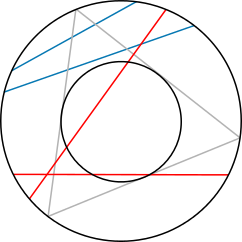Random radial points, selection method 2; red = longer than triangle side, blue = shorter

In this method, we consider two equilateral triangles ABC and EFG that are drawn upside down. When you draw a circle that makes perfect contact with the internal sides of these two triangles, we conclusively infer that, any random chord you draw that passes through these inner circle drawn, the length of such chord is always longer than the length of the sides of the equilateral triangle.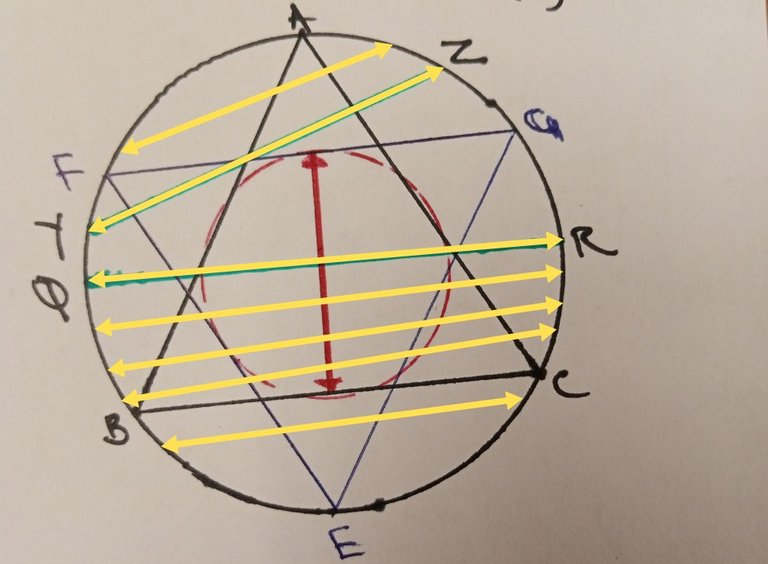If you take a careful look at the diagram I have sketched above, you would observe that the lengths QR which falls within the inner red circle is longer than the length of YZ. By virtue of this, all chords within the inner circle are all longer than the length of the sides of the triangles. It does not matter how you draw it, provided the chord passes through the center of the smaller circle, it will always be longer than the lengths the triangle and also lengths of those chords that do not pass through the inner circle. So then how do we calculate the probability of this?

Well the approach to this also has various methods. For those that understand geometry well, this would sure be a cake walk. However, I am not a mathematician, so I will also give you the best and most simple approach which I find more comfortable to work with. Alright, let's get to it!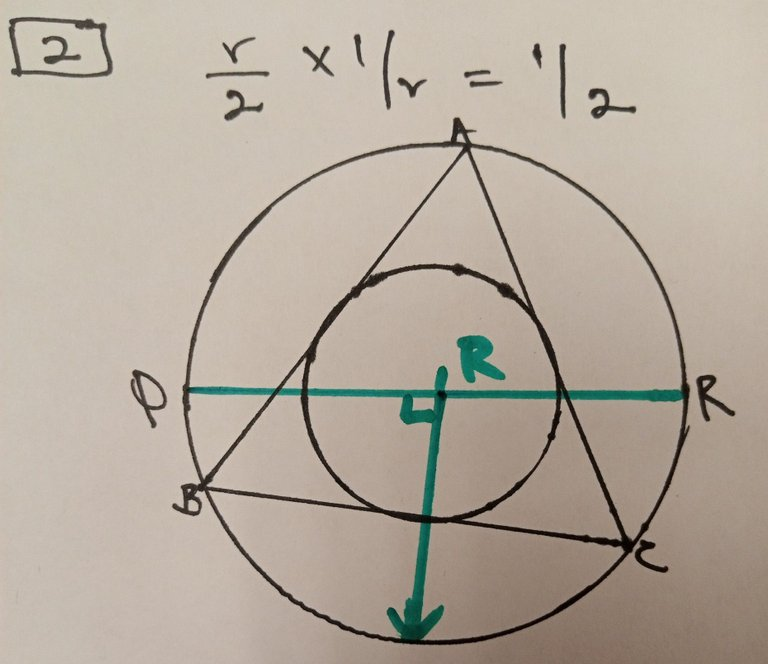Since we already know that the chord say QR lands or passes within the inner circle is longer than the triangle as can be seen the images I have clearly drawn, then if we are to look at the probability of the chord being within the smaller circle or the random radius being inside the smaller circle, then the only possible way for this is R/2 while the total possible ways we can do it will be R.

The is so because the total radius is R (the fixed radial line) while possible outcome is just half of this radius hence, R/2. Similarly, applying the classical probability approach, we obtain R/2 / R = 1/2. You can as well apply the Pythagoras formula by drawing a line from the center of the inner circle to point G foe example and name this line R. Solving the arithmetic should still land you to 1/2. The last way of drawing the chord is the Random midpoint method.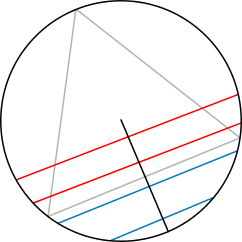Random chords, selection method 3; red = longer than triangle side, blue = shorter

This method is just similar with the random radial method we just discussed because we are still considering the the inner circle within the equilateral triangle. Similarly, the length of any chord that passes through the inner circle is always longer than the lengths of the sides of the triangle. The only difference lies in the approach we use in calculating the probability that the length of the chords will be longer than the sides of the triangle.

Here we consider the area of the two circles, the bigger and the smaller (inner) one. The area of a circle is given as πr2. So having this in minds, applying the classical probability again, we have Area of small circle / Area of Big circle. Where the radius of the big circle is assumed 1, while that of the smaller circle is just half, 1/2 of the bigger circle.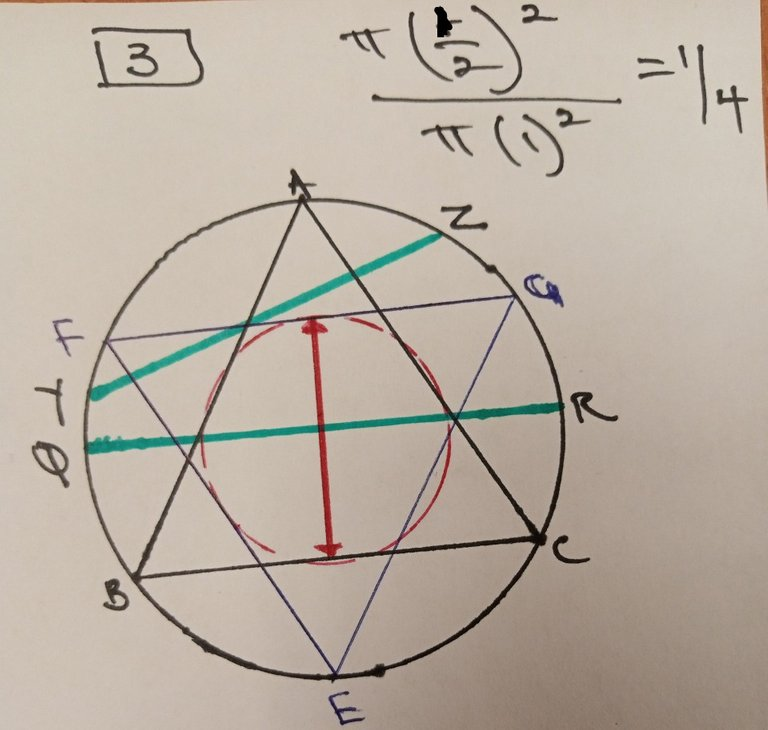We then have πr2/πr2, inserting the values of the radius for the smaller and big circle, we arrive at (1/2)2 / (1)2. Doing the simple arithmetic gives us 1/4.

With all the three approaches we have discussed, it is possible to have to draw random chords that are longer than the lengths of the sides of an equilateral triangle inscribed in a circle.

In all, the take home from this experiment is that the paradox arises because it is not immediately clear which method is the "correct" one to use. Each of the method and approach to solve it seems reasonable, however, they lead to different probabilities. In fact, the paradox highlights the importance of carefully defining the method for selecting random points on a geometric object before computing probabilities. Clearly defining your experiment matters in this sense. I hope it all makes a whole lot of sense to you. I want to believe it does.

Alright, that will be all for today. Busy days are gradually encroaching, as a result, my activities on-chain will soon once again gradually decline. Enjoy the rest of your day.

0
0
0.000This post has been given a 7.5% UpVote by the SplinterBoost Community Curation Bot.Vote For Witness | Delegate HP | Join Discord

0
0
0.000### Congratulations!### ✅ Good job. Your post has been appreciated and has received support from CHESS BROTHERS ♔ 💪

♟♟ You can also reach us on our Discord server and promote your posts there.

♟♟♟ Consider joining our curation trail so we work as a team and you get rewards automatically.

♞♟ Check out our @chessbrotherspro account to learn about the curation process carried out daily by our team.

🥇 If you want to earn profits with your HP delegation and support our project, we invite you to join the Master Investor plan. Here you can learn how to do it.

Kindly

The CHESS BROTHERS team

0
0
0.000Thanks for your contribution to the STEMsocial community. Feel free to join us on discord to get to know the rest of us!

Please consider delegating to the @stemsocial account (85% of the curation rewards are returned).

Thanks for including @stemsocial as a beneficiary, which gives you stronger support.

0
0
0.000